# H.C.F concepts and formula

## H.C.F concepts and formula

One of the most prevalent quantitative aptitude topics asked in government tests is H.C.F This is one of the most fundamental topics that students are already aware of before they begin studying for competitive exams.

Although the concepts of H.C.F remain the same, the kind of questions presented in exams may vary. H.C.F is used in all the topic of Quantitative Aptitude, this makes this topic very important.

2-3 word problems related to H.C.F are asked in exams, but candidates may also expect questions about data sufficiency and data interpretation related to H.C.F topic.

So, we are providing you with concepts and formulas for the H.C.F topic to help you prepare properly for the upcoming exams.

When we travel from one point to another, there will be three Quantity that defines this journey – H.C.F.

## What is H.C.F

The highest common factor (HCF) of two whole numbers is the largest whole number which is a factor of both. Or HCF of the two numbers is the highest number of the two numbers which divides them exactly.

Consider the numbers 12 and 15:

The factors of 12 are : 1, 2, 3, 4, 6, 12.

The factors of 15 are : 1, 3, 5, 15.

1 and 3 are the only common factors (numbers which are factors of both 12 and 15).

Therefore, the highest common factor of 12 and 15 is 3.

## Properties of HCF

HCF of two or more numbers is the divides the given numbers, exactly

HCF of prime numbers is always equal to 1

HCF is always less than or equal to the given numbers

## How to Calculate  HCF?

• by prime factorization method: Break the given numbers into prime factors and then find the product of all prime factors common to all the numbers. The product will be the required HCF.
• by division method : Divide the greater number by smaller number, divide the divisor by the remainder, and divide the remainder by the next remainder, and so on until no number is left. The last divisor is the required HCF.

### HCF By Prime Factorization Method

Step 1: Write each number as a product of its prime factors. This method is called here prime factorization.

Step 2: Now list the common factors of both the numbers

Step 3: The product of all common prime factors is the HCF ( use the lower power of each common factor)

Let us understand with the help

Example 1  Find the HCF of 60 and 45.

Prime factor of 60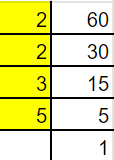60 = 2 x 2 x 3 x 5

Prime factor of 45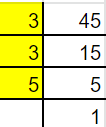45 = 3 x 3 x 5

HCF = 3 x 5 = 15

Example 2 : Find the HCF of 36, 24 and 12.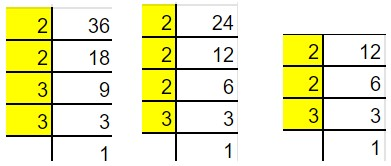Prime factor of

36 = 2 x 2 x 3 x 3
24 = 2 x 2 x 2 x 3
12 = 2 x 2 x 3

HFC = 2 x 2 x 3 = 12

### H.C.F. by division method

Step 1: Divide larger number by smaller number first, such as; Larger Number/Smaller Number
Step 2: Divide the divisor of step 1 by the remainder left. Divisor of step 1/Remainder

Step 3: Again divide the divisor of step 2 by the remainder. Divisor of step 2/Remainder

Step 4: Repeat the process until the remainder is zero.

Step 5: The divisor of the last step is the HCF.

Example 1 Find the H.C.F. of 12 and 18.HCF = 6

### HCF by short division method.

Step 1: Write the given numbers horizontally, in a sequence, by separating it with commas.

Step 2: Find the smallest prime number which can divide the given number. It should exactly divide the given numbers. (Write on the left side).

Step 3: Now write the quotients.

Step 4: Repeat the process, until you reach the stage, where there is no coprime number left.

Step 5: We will get the common prime factors as the factors in the left-hand side divides all the numbers exactly. The product of these common prime factors is the HCF of the given numbers.

Example 2:  Find the HFC of 18 and 54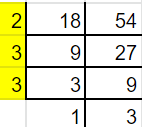HCF = 2 x 3 x 3 = 18

Example 2: Find out HCF of 36, and 24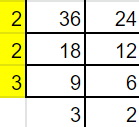HCF = 2 x 2 x 3 = 12

## How to find the HCF of Three numbers

To find the highest common factor of three numbers by using division method is discussed here step by step.

Step I: First of all find the highest common factor (H.C.F) of any two of the given numbers.

Step II: Now find the highest common factor (H.C.F) of the third given number and the highest common factor (H.C.F) obtained in Step 1 from first and the second number.